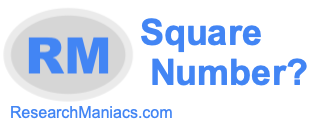Is 8 a square number?Here we will answer the question: "Is 8 a square number?" You could also ask "Is 8 a perfect square?" Square number and perfect square mean the same thing.

Anyway, for 8 to be a square number, 8 must be the product of an integer multiplied by itself. In other words, the square root of 8 must equal a whole number (integer).

There is no integer that you can multiply by itself that will make 8. Furthermore, the square root of 8 is not an integer. Thus, 8 is NOT a square number.

The answer to the question: "Is 8 a square number?" is No.
The answer to the question: "Is 8 a perfect square?" is also No.

Square Number?
Enter another number to see if it is a square number:

Is 9 a square number?
Find out if the next number on our list is a perfect square.